# Shift Micro-Operations in Computer Architecture

• Difficulty Level : Easy
• Last Updated : 16 Oct, 2020

Shift micro-operations are those micro-operations that are used for serial transfer of information. These are also used in conjunction with arithmetic micro-operation, logic micro-operation, and other data-processing operations.

There are three types of shifts micro-operations:

Attention reader! Don’t stop learning now.  Practice GATE exam well before the actual exam with the subject-wise and overall quizzes available in GATE Test Series Course.

Learn all GATE CS concepts with Free Live Classes on our youtube channel.

1. Logical :
It transfers the 0 zero through the serial input. We use the symbols shl for logical shift-left and shr for shift-right.

1. Logical Shift Left –
In this shift one position moves each bit to the left one by one. The Empty least significant bit (LSB) is filled with zero (i.e, the serial input), and the most significant bit (MSB) is rejected.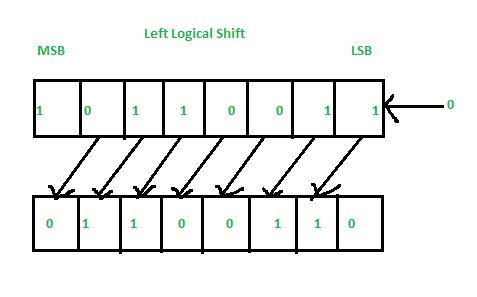2. Right Logical Shift –
In this one position moves each bit to the right one by one and the least significant bit(LSB) is rejected and the empty MSB is filled with zero.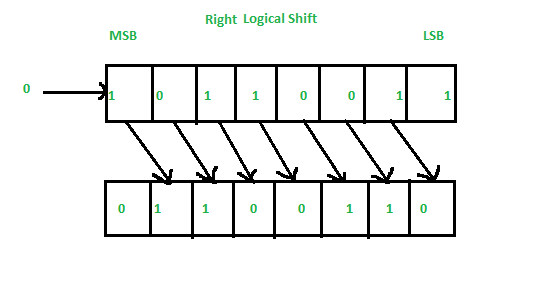2. Arithmetic :
This micro-operation shifts a signed binary number to the left or to the right position. In an arithmetic shift-left, it multiplies a signed binary number by 2 and In an arithmetic shift-right, it divides the number by 2.

1. Left Arithmetic Shift –
In this one position moves each bit to the left one by one. The empty least significant bit (LSB) is filled with zero and the most significant bit (MSB) is rejected. Same as the Left Logical Shift.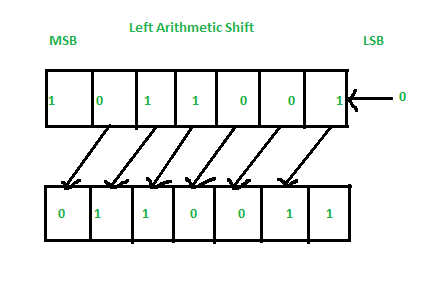2. Right Arithmetic Shift –
In this one position moves each bit to the right one by one and the least significant bit is rejected and the empty MSB is filled with the value of the previous MSB.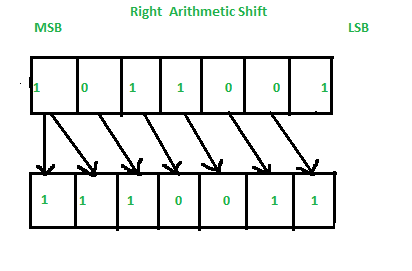3. Circular :
The circular shift circulates the bits in the sequence of the register around the both ends without any loss of information.

1. Left Circular Shift –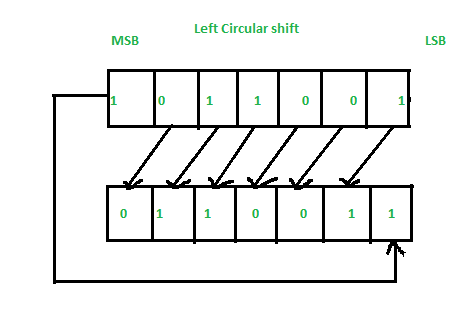2. Right Circular Shift –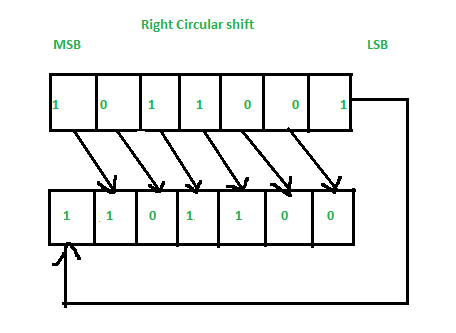My Personal Notes arrow_drop_up